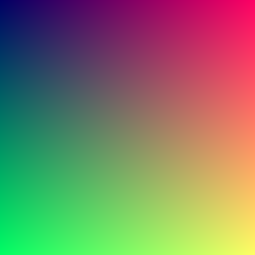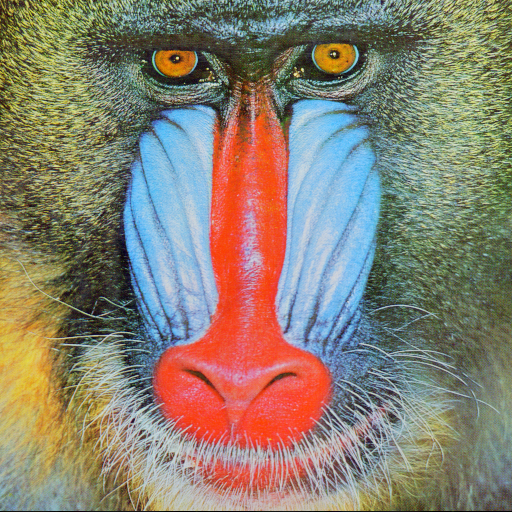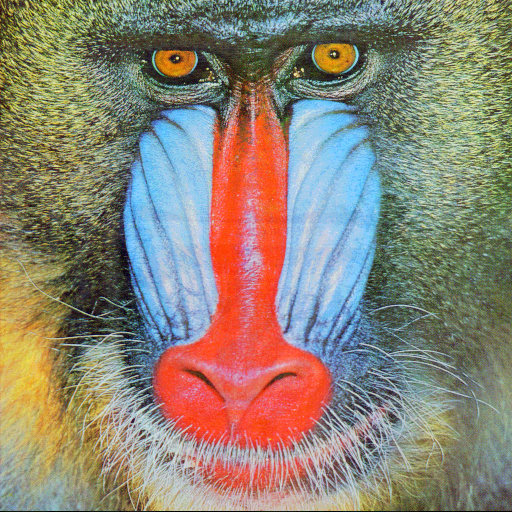## Steganography, Python and Image manipulation with Pillow

Recently at Uni I got a math class about number bases, one of the things they mentioned in the class was Steganography and I was a little curious about it and found the Steganography page in Wikipedia. Oh boy, so many things made sense to me!

While steganography is not just related to images, in this blog post I will concentrate in its use with images, the idea is simple: We can put another image or file inside an existing image without affecting (too much) the original image.

How? well, given an image is a set of pixels and each pixel is represented by a coordinate (x, y) with a color, and we represent color with a tuple with the intensity of three colors (red, green, blue), each color goes from 0 to 255 and we can represent that number as a 8 digit binary number, for example, $$192_{10} = 1100 0000_{2}$$. Now, the most significant bits in that number are $$1100$$ and the rest ($$0000$$) doesn’t contribute that much to the information, so they are called less significant bits (this could be the relevant article in Wikipedia about it).

Let’s move to Pillow. Pillow is a friendly fork of of PIL, it is super easy to use and support many image file formats and operations (you can even extend it to support your own crazy file format). Using Pillow as a virtual canvas to draw using pixels is easy:

from PIL import Image

colorful = Image.new('RGB', (255, 255), 'black')

for x in range(0, colorful.width):
for y in range(0, colorful.height):
colorful_pixels[x, y] = (x, y, 100)
colorfulWe can read an existing image and get not only image but pixel information as well:

baboon = Image.open('baboon.png')
print(baboon.width, baboon.height)
print(baboon_pixels[312, 216])
baboon

Out[*]:
512, 512
(181, 200, 220)Ok, let’s return to steganography. Let’s say we have the image of this airplane:

airplane = Image.open('airplane.png')
airplaneAnd we want to hide that image in the image of our previous baboon. How do we do this? well, remember our talk about LSB and MSB? the idea is taking the MSB from the image to hide and replace the LSB of the cover up image with them, at the end we should generate an image very similar to our baboon but hiding the airplane!

As I mentioned before, the idea is very simple, we take the image we want to hide (let’s call it secret) and read the color channel information for a given pixel, let’s say, pixel (312, 216) has color information (53, 31, 109). Now, we read the same color info from the image we will use as cover up, (181, 200, 220). Time for the interesting part, we take the color info one by one, convert them into binary, $$181_{10} = 1011 0101_2$$ for the baboon red channel pixel (312, 216) and $$53_{10} = 0011 0101_2$$ for the same airplane image pixel, now we take the most significant 4 bytes from the airplane (secret) image and place them instead of the less significant 4 bytes of the baboon image (cover up), resulting $$1011 0011_2 = 179_{10}$$ for the same pixel, notice even in decimal there is no much distance or difference between the original color channel for red ($$181_{10}$$) and the resulting secret ($$179_{10}$$), we repeat this process for each pixel and color channel and that’s all!.

There are many ways to do this in Python, but for simplicity we will use the long way, converting the number to string using Python format strings, extracting 4 left and 4 right bytes for msb and lsb and then joining them as one binary number for later converting back into integer using intspecifying base 2. Let’s see that in code!

cover_up = Image.new('RGB', (baboon.width, baboon.height), 'black')

for x in range(0, cover_up.width):
for y in range(0, cover_up.height):
br, bg, bb = baboon_pixels[x, y]
ar, ag, ab = airplane_pixels[x, y]

# first 4 bytes from cover up (msb) +  first 4 bytes of secret
cr = int(f'{br:08b}'[:4] + f'{ar:08b}'[:4], 2)
cg = int(f'{bg:08b}'[:4] + f'{ag:08b}'[:4], 2)
cb = int(f'{bb:08b}'[:4] + f'{ab:08b}'[:4], 2)

cover_up_pixels[x, y] = (cr, cg, cb)
cover_upYou could argue the baboon image is not exactly the same as the original image, but it is a good approximation (remember, we lost color channel information in the process), but at the naked eye you cannot tell me there is an airplane hidden in the same image!.

Let’s extract now the secret image from the cover up, the process is kind of the opposite, we know the most significant bytes of our secret are hidden in the less significant bytes of our cover up, and then we just complete the lsb with zeroes.

secret = Image.new('RGB', (cover_up.width, cover_up.height), 'black')

for x in range(0, secret.width):
for y in range(0, secret.height):
r, g, b = cover_up_pixels[x, y]

sr = int(f'{r:08b}'[4:] + '0000', 2)
sg = int(f'{g:08b}'[4:] + '0000', 2)
sb = int(f'{b:08b}'[4:] + '0000', 2)

secret_pixels[x, y] = (sr, sg, sb)
secretThere it is! This technique could be implemented to hide any type of file inside another, for example, I remember long time ago it was common to hide RAR files inside GIF images. Wikipedia even mention some printer manufacturers use them as a way to print and identify printers by their serial number for many years without anybody ever notice it.

Go and play with it, I really enjoyed it :)